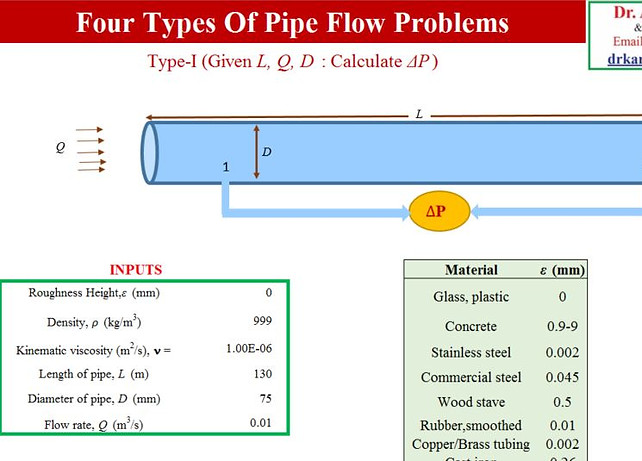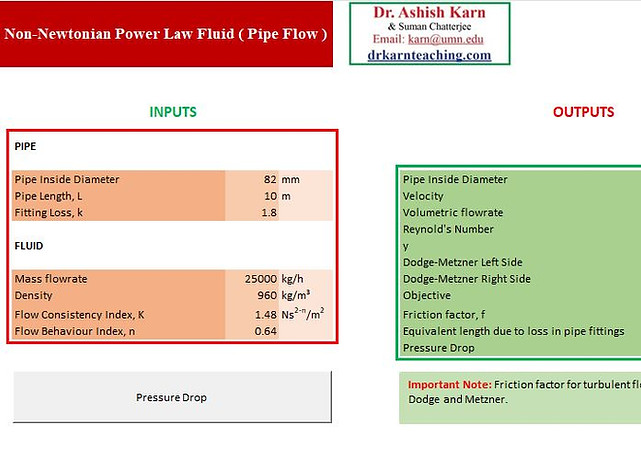top of page
##### Fluid Mechanics Tools

Visualization of manometer by changing parameters.Visualization of pitot tube by changing manometric height and velocity of fluid.Visualization of change in capillary height as the values of surface tension, angle of contact, density and capillary radius changes.Visualization of buoyancy on a floating cylinder.Here is a computerized version of the Moody Chart, which can prove very useful in the determination of Darcy friction factors for a variety of pipe sizes and materials with different roughness heights. Darch friction factor is crucial in pressure drop calculations for turbulent pipe flows. This Moody chart solver solves implicit Colebrook Equation and shows a graphical visualization as well. Click on the title to download.This small tool allows you to calculate different fluid properties, such as dynamic viscosity, density, kinematic viscosity, specific heat, thermal conductivity, thermal diffusivity of a variety of fluids at a given temperature. It also provides Prandtl number and Reynolds number (provided velocity and length scales are provided).Solutions to Four types of Pipe Flow ProblemsSolutions to equivalent length of pipe required after removing all the valves and fittings.Visualization of Couette flow by changing parameters.Visualization of double sided Couette flow by changing parameters.Visualization of Betz's equation with the help of graph.Visualization of 2-D flow field by changing parameters.Visualization of volumetric flow rate as the height of liquid in tank changes.Visualization of lift and drag coefficients by changing parameters.Here is an interactive tool that computes the anchor force on an aircraft test engine because of the momentum changes between inlet and outlet. The users can vary not only the inlet velocity (i.e. the aircraft speed), but also the area of the inlet as well as the outlet section. Similarly, the gage pressure at inlet and outlet sections can also be entered, and the corresponding changes in the anchor (/thrust) force observed.Visualization of different isobaric surfaces when a fluid is rotating in a cylinder.Visualization of pressure change as the height of different fluid layer changes.Visualization of forces on a partially submerged surface due to water filled in tank.Visualization of forces on a completely submerged surface due to water filled in tank.Visualization of different forces on a kite and tension in string.Calculation of pressure drop for a Non-Newtonian Power Law fluid for a pipe flow.Calculation of pressure drop for a Non-Newtonian Bingham plastic fluid for a pipe flow.Calculation of pressure drop for a two phase flow in a horizontal pipe using Friedel's correlation.Calculation of throat velocity and mass flow rate for a flow through a venturi.bottom of page